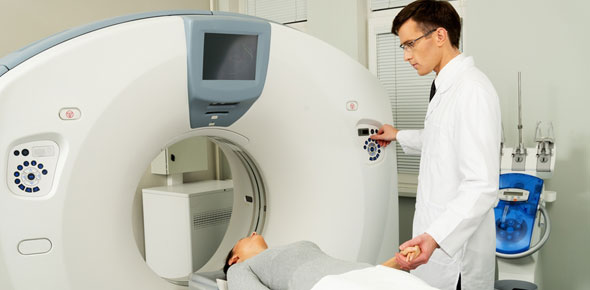# CT Registry Physics Hhh

14 Questions | Total Attempts: 2712SettingsUnderstanding physics for ct registry

• 1.
The full width at half maximum (FWHM) of a CT scanner is used to describe:
• A.

Spatial resolution

• B.

Contrast resolution

• C.

Noise

• D.

Calibration accuracy

• 2.
Voxels with equal dimensions along the x, y, and z axes are referred to as
• A.

Prospective

• B.

Isotropic

• C.

Retrospective

• D.

Anisotropic

• 3.
The average photon energy of the primary beam of a CT scanner operating at a tube potential of 120 kVp is approximately
• A.

50 keV

• B.

70keV

• C.

100 keV

• D.

120 keV

• 4.
The acquisition of a series of CT images at a single anatomic location over a set period is referred to as;
• A.

Ultrafast ct

• B.

Cine ct

• C.

Conventional ct

• D.

Temporal ct

• 5.
The rate at which  a quantity of x-radiation emitted from a CT tube passes through a unit area over a unit of time is called the:
• A.

Effective mAs

• B.

Photon flux

• C.

Constant mAs

• D.

Photon fluence

• 6.
A standard CT image reconstructed on a 512 matrix has a digital size of approximately
• A.

0.5 mb

• B.

3.0 MB

• C.

5.0 mb

• D.

10.0 mb

• 7.
The 3d ct technique that includes all of the acquired voxel information in the reconstructed model with adjustments to its opacity is termed;
• A.

Surface rendering

• B.

MIP

• C.

Curved multiplanar reformation

• D.

Volume rendering

• 8.
The contrast resolution of a cT scanner is not related to which of the following
• A.

Focal spot size

• B.

Section width

• C.

Reconstruction algorithm

• D.

Signal-to-noise ratio

• 9.
The average photon energy of the ct x-ray beam can be increased by
• A.

Increasing mAs

• B.

Increasing filtration

• C.

Increasing collimation

• D.

All of the above

• 10.
The type of MSCT detector array that contains multiple rows of detector elements, each of the same length, is called
• A.

Uniform matrix array

• B.

• C.

Hybrid array

• D.

Stationary array

• 11.
Reformatted ct image planes that lie perpendicular to the original plane of acquisition may be described as
• A.

Oblique

• B.

Orthogonal

• C.

Obtuse

• D.

Orthographic

• 12.
Segmenting the data acquisiton process into separate componenets of smaller rotation angles may improve which of the following componenets of CT image quality
• A.

Temporal resolution

• B.

Longitudinal spatial resolution

• C.

Contrast resolution

• D.

In-plane spatial resolution

• 13.
Which of the following image artifacts is unique to multislice CT (MSCT) systems
• A.

Step artifact

• B.

Aliasing artifact

• C.

Out of fild artifact

• D.

Cone beam artifact

• 14.
A 16-slice MSCT system utilizes an adaptive array of 24 detectors, each ranging in size from 0.625 to 1.25 mm What is the maximum number of sections the system can acquire with each rotation of the gantry
• A.

8

• B.

16

• C.

32

• D.

64

Related TopicsBack to top